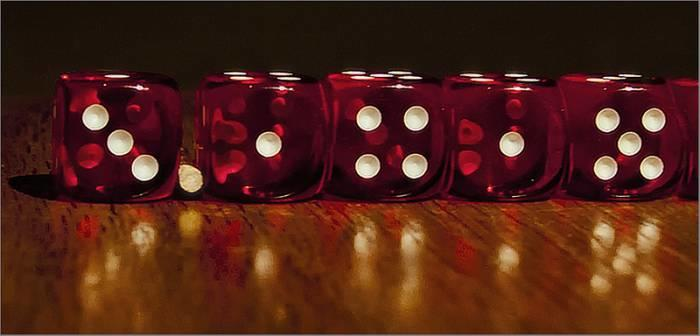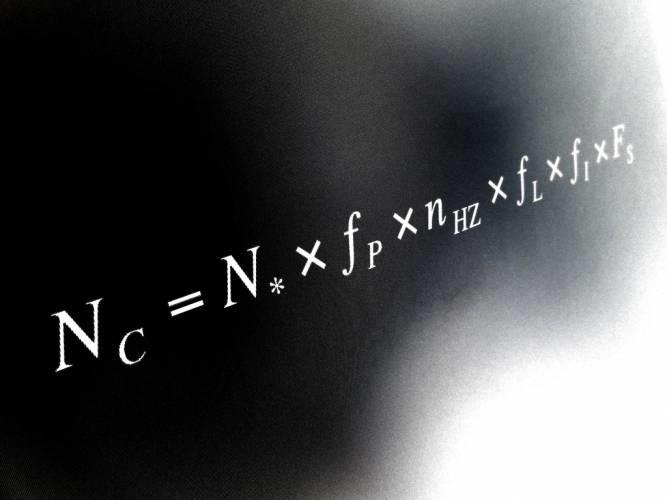Share

Mathematical Mysteries Unveiled

By Shreyanjana, published on 19/09/2019 > > > Mathematical Mysteries of the Universe

“Mathematics is an inescapable part of our everyday life experiences. And yet, very few people are aware of this.”

Mathematics is a lot more than multiplication, division, fractions, algebra, geometry or the several other nightmarish functions that are taught to us in school and which most of grow to hate! The subject matter of mathematics, in many ways, contains the essence of the world we inhabit. And, the day you realize this, you’ll be grateful to your math teacher (perhaps).

Indeed, mathematics is nothing short of an enigma, the mysteries to which the world of science is still unravelling. Endless debates on the creation or the discovery of mathematics, impossible or unsolved equations, a fascination with certain numbers, unusual formulas, and so on have all contributed to creating a sort of reverence towards the subject, no matter how much we dislike studying it for the exams.

Staying on the topic of reverence, you will be surprised to discover how many mathematical concepts and theories are based or have their origins in religio-mythological beliefs which have then been incorporated into the scientific mainstream.

Close your eyes for a moment and try to imagine infinity. Just like the idea of God, infinity cannot be seen nor touched. But, there is a belief that it exists. To the ancient Mayans, math and religion seemed one.

Mathematics: The Beauty and Magic of Numbers

“Here is some food for thought: was mathematics discovered or is it a natural occurrence?”

Math: A Natural Abacus

The history of mathematics shares its antiquity with the history of humanity. From the notched artefacts of early human settlements to the agrarian civilizations of Mesopotamia, Indus Valley and Egypt, from the progressive thinking of the earliest Greek city-states to the mighty Hellenistic kingdom, mathematics has had a glorious journey through time.

Equipped with mathematical knowledge, scientists throughout history have made remarkable discoveries. Examples include the decoding of planetary orbits, discovery of Higgs boson, landing of the Curiosity Rover on Mars, to name only a few.Landing a robot on Mars? It’s easy peasy with mathematics! Source: visualhunt

India’s Unmistakable Contributions to the World of Mathematics

The Indian subcontinent, as we all are aware, is steeped in history, some of them indeed remarkable. It has made some invaluable contributions to the enrichment of mathematical studies throughout history.

The Harappan Decimal System

Among the rich haul of artefacts from Harappan sites are weights corresponding to ratios of 0.05, 0.1, 0.2, 0.5, 1, 2, 5, 10, 20, 50, 100, 200, and 500 along with scales with decimal divisions. These earliest artefacts depicting the decimal system are remarkably accurate!

The Vedic Number System

The Vedic texts contain mathematical knowledge in the form of numbers expressed in combinations of powers of ten. Brahmi numerals make their appearance in the 3rd century BCE.

Zero

Zero has a complicated history, first recorded, as it were, in Babylonian and Sumerian manuscripts as simple placeholders. But, it was only in the 6th century CE India that the placeholder zero became a number in its own right!

Astronomer Brahmagupta is credited with the introduction of solutions to quadratic equations and computation of square roots in his seminal work, Brahmasputha Siddhanta.

The First Calculus

Aryabhatta, the celebrated scientist from the Vedic age is credited with the introduction of the concept of infinitesimals or tatkalika gati. He used it to precisely map the lunar eclipse and expressed it in the form of a basic differential equation.

Paying Homage

“The documentary, “The Great Math Mystery” (2015) is a good starting point if you are looking to trace the philosophical debates about the universality and effectiveness of this great discipline.”

The documentary follows math wizards across history, including Pythagoras, Galileo, Newton and Einstein, and their fascinating discoveries, once topics of heated philosophical and even religious debates, but now considered scientific dogma. The documentary reinforces the enigmatic nature of Mathematics that is ever-growing.

The Unsolvable Math Mysteries and Puzzles

“Did you know that there are still 7 mathematical problems that have never been solved?”

These have been together dubbed as the 7 mathematical problems of the millennium.

The quest to find solutions to the ‘unsolvables’ in mathematics started in 1900 when mathematician D.Hilbert drew up a list of 23 unsolved mathematical problems. A century later, in 2000, mathematicians were able to solve the majority of the 23. However, 7 still remain unsolved.

To support research into these scientific mysteries, an American billionaire, L. Clay, set up the CMI (Clay Mathematics Institute) in 1999. He announced a reward of £1 million to anyone who took up the challenge and succeeded at solving any one of these ultimate mathematical problems!

“Think you are up for the challenge? Find out more about it here.”A million pounds to solve an equation? Does anyone have a calculator? | Source: visualhunt

Here are the 7 Millennium Problems (without their solutions, of course).

• The Riemann hypothesis
• The Hodge conjecture
• The Birch and Swinnerton-Dyer conjecture
• The Navier-Stoke equations
• The Yang-Mills Equations
• P versus NP
• Poincaré conjecture

Very few mathematicians in the world have the opportunity to work on these equations and conjectures. Work harder on your math and ace those tests at school and university.

As Albert Einstein says:

“We cannot solve our problems with the same thinking we used when we created them.”

Wise words from a mad genius!

Do you know of any mathematical mysteries and paradoxes that are yet to be decoded?

The Most Loved Numbers of the World

Yes, you read that right. There are people who love numbers and who worship mathematics. We are hoping, after reading this article, you will develop some form of affection towards the subject.

Pi: The Most Secretive Number

Pi is perhaps the most well-known mathematical constant.

It represents the ratio between the circumference of a circle and its diameter. Its approximation stands at 3.14 or 22/7.

1. Pi is an irrational number. It cannot be written as a fraction and its decimal places are infinite.  he most approximate decimal value of Pi, calculated till date, has a whopping 1,241 billion digits. It was calculated by an extra-powerful computing software.
2. Pi is a transcendental number, which means it cannot be the solution to any polynomial equation with integer coefficients.
3. Mathematicians are still debating whether Pi is a normal number. In other words, it is still a matter of conjecture if Pi can have a finite sequence of decimal places.
4. The value of Pi is an approximation but, paradoxically, it allows us to make extremely accurate calculations.
5. Pi would equal the ratio between the perimeter of the base of a pyramid and double the height.How many numbers of Pi can you remember? | Source: visualhunt

Phi: Not to be confused with Pi

“Phi, otherwise known as the golden number, has a mythical origin.”

Phi, also called the Golden Ratio, is a special number obtained by dividing a line into two parts so that the longer part divided by the smaller part is also equal to the whole length divided by the longer part. Mathematicians suggest that Phi could be present in all things in our universe – spaces, plants, and even human beings.

Phi is the solution to a quadratic equation. Closely linked to the famous Fibonacci sequence, the law of Phi is also referred to as the “divine proportion”. The “Vitruvian Man” by celebrated Renaissance artist-scientist-philosopher, Leonardo da Vinci, is one of the most thought-provoking examples of the law of Phi.

“If you measure the distance between the ground and the top of your head and divide it by the distance between the ground and your navel, you will get…wait for it…The value of Phi , about 1.618.”

Se7en

The number 7 has not only invoked terror in the hearts of movie buffs who have watched this Brad Pitt Kevin Spacey thriller but has, more importantly, been present in many of our cultural contexts, past and present. Some examples include:

• The 7 Wonders of the World;
• The 7 colours of the rainbow;
• The 7 days of the week.

Not surprisingly, the number 7 is also significant in basic mathematics and geometry. Consider these rules:

• 7 acute triangles divide into an obtuse triangle,
• The 7th is omnipresent in the triplets of Pythagoras,
• 7 types of “disaster” models in mathematics,
• 7 is a magic number,
• 7 is a prime number, and so on.

To this day no scientist has come up with a real explanation for the strong presence of the number 7 in mathematics. A mystery, a symbol, a myth … Everyone is free to make their own interpretation.

“Is 7 your lucky number? You might want to check with your family astrologer!”

Drake’s Mysterious Equation

Drake’s Equation, named after its creator, Frank Drake, was developed to determine the number of “possible” intelligent civilizations in our galaxy. The Equation has been used by Exobiologists, Futuro-biologists, Astro-sociologists as well as the SETI (Search for Extra-Terrestrial Intelligence) project.

Published in 1961, Drake’s formula still divides scientists and mathematicians. No known scientist or anyone, for that matter, possess the necessary knowledge to fully decipher the Equation.Drake’s mathematical equation. | Source: visualhunt

“Do you think we are alone in this universe? Will we ever know who is watching us from outer space? That is indeed a very unsettling thought!”

Mathematics – More Than A Mere Subject

Mathematics has a lot to offer young minds that are an ocean of curiosity. With newly developed educational resources, there is a renewed interest in mathematics in Indian schools; teachers are trying to make math learning fun through math games, logic games, magic calculations, etc.

Recently, there has been a major experiment in mathematical networks theory that has once again proven the unmistakable presence of math in culture, including popular culture. Find out how two passionate mathematicians used their knowledge to discover the main character in Game of Thrones.

India needs to rethink its math curriculum and break away from the “one size fits all” mantra. Introducing different levels of mathematics from Class IX onwards seem highly practical and a good starting point as anxiety levels rise among students with the impending Board exams.

Share(No ratings so far)Loading...